Mobile QR Code1. (Department of Electronic Engineering, Hanyang University)

## I. INTRODUCTION

The signal-to-quantization noise ratio (SQNR) of a delta-sigma ADC can be improved by increasing the over-sampling ratio (OSR) or the order of the loop filter [1-3]. Multi-stage noise shaping (MASH) is a method to increase the order of the noise shaping without worsening the instability caused by higher order loop-filters [1,4,5]. In a two-stage MASH structure, for example, the quantization error of the first stage is quantized by the second stage. Then, the digital output of the second stage undergoes a digital filtering, of which the transfer function (TF) is identical to the noise transfer function (NTF) of the first stage. The final output, which is obtained by subtracting the filtered second stage output from the first stage output, has only the quantization noise of the second stage, which can be made much smaller than that of the first stage.

A challenge in the design of a MASH type delta-sigma ADC is to generate an analogue representation of the quantization noise of the preceding stage and pass it on to the next stage to be quantized again. The most straightforward way is to use a digital to analog converter (DAC) and an analogue subtractor. However, this method is prone to error, which leads to a leak of the first stage quantization noise to the final output.

Recently, a 2-stage MASH structure, which used a single successive approximation register (SAR) ADC for both the first stage and the second stage quantizer, was proposed . In the MASH ADC, the quantization error of the first stage was automatically generated and passed on to the next stage in the natural operation of the SAR ADC, which greatly simplified the design of the two-stage ADC.

Now, the most serious issue remaining in the design of the MASH ADC is the mismatch between the TF of the digital filter acting on the second stage output and the NTF of the first stage caused by finite gain of the op-amps in the loop filter. When there is mismatch between the TFs, the cancellation of the first stage quantization noise becomes imperfect and the final SQNR is degraded. The simplest way to prevent the SQNR degradation from the mismatch is to use op-amps with a high gain. However, it becomes more difficult to design high-gain op-amps as the supply voltage of CMOS circuit is reduced. Therefore, it is meaningful to find a way to lower the minimum op-amp gain requirement in the design of the MASH ADC.

In this paper, we show that the use of correlated double sampling (CDS) integrators [8,9] along with the loop-gain adjustments can greatly reduce the SQNR degradation from the NTF mismatch and can lower the minimum op-amp gain to a very low level. The rest of this paper is organized as follows. In Section II, we present the MASH ADC which uses a single SAR ADC quantizer. In Section III, we analyze the SQNR performance of the ADC considering the TF mismatch from finite op-amp gain and demonstrate that significant reduction of the SQNR degradation from the finite op-amp gain is possible by employing CDS integrators along with the adjustment of the quantizer gain. In Section IV, we conclude.

## II. THEORY

Fig. 1. Block diagram of the MASH ADC studied in this work.Fig. 1 shows a block diagram of the MASH type delta-sigma ADC. The SAR ADC functions as the quantizer for both the first and the second stages. Of the $N_{SAR}$-bit output of the SAR ADC, $N_{SAR}$ most significant bits (MSBs) are used as the first stage output ($V_1$) and $N_{LSB}$ = $N_{SAR}$ – $N_{SAR}$ least significant bits (LSBs) are used as the second stage output ($V_2$). Note that after $N_{SAR}$-bits have been resolved, the quantization error $E_1$ is automatically generated in the operation of the SAR ADC. Then, the subsequent operation of the SAR ADC can be understood to be that of the second stage quantizer acting on $E_1$. $H_1$ and $H_2$ are the digital filters acting on the first and the second stage outputs, respectively. $L_0$ and $L_1$ represent the first stage loop-filter functions for the input ($U$) and the feedback signal ($V_1$), respectively. It is well known that the NTF and signal transfer function (STF) of the first stage can be represented by $NTF_{1}=1/(1+L_{1})$ and $STF_{1}=L_{0}/(1+L_{1}),$ respectively, and the final output can be expressed by

##### (1)
\begin{equation*} \begin{array}{c} V\left(z\right)=H_{1}\left(z\right)\left[STF_{1}\left(z\right)U\left(z\right)+NTF_{1}\left(z\right)E_{1}\left(z\right)\right] \end{array} \end{equation*} $+H_{2}(z)\left[E_{2}\left(z\right)-E_{1}(z)\right]$.

where $E_2$ is the quantization error of SAR ADC after resolving whole $N_{SAR}$ bits. In (1), if $H_2$ = $H_1$${\cdot}$$NTF_1$, two terms containing $E_1$ cancel each other and the output becomes

##### (2)
$V\left(z\right)=H_{1}\left(z\right)\left[STF_{1}\left(z\right)U\left(z\right)+NTF_{1}\left(z\right)E_{2}\left(z\right)\right].$

In (2), $V(z)$ contains only the quantization noise of the second stage $E_2$, of which the power is smaller than that of $E_1$ by a factor of $2^{{2N_{LSB}}}.$ In (1), if there is mismatch between $H_2$ and $H_1$${\cdot}$$NTF_1$, the cancellation of $E_1$ becomes imperfect, and the leaked $E_1$ degrades the final SQNR.

A main cause of the mismatch is finite gain of the op-amps used in the integrators. Fig. 2 shows schematics of switched-capacitor (SC) integrators having nominal gain of $d_0$ = C$_{1}$/C$_{2}$. In Fig. 2, ‘1’ and ‘2’ indicate two non-overlapping clock phases. Fig. 2(a) shows a conventional integrator and Fig. 2(b) shows a CDS integrator . The TF of the integrators can be expressed as

##### (3)
\begin{equation*} \begin{array}{c} I\left(z\right)\equiv \frac{V_{out}}{V_{in}}\left(z\right)=\frac{dz^{-1}}{1-pz^{-1}}. \end{array} \end{equation*}

where $d$ is the gain and $p$ is the pole of the integrator TF. If the op-amp gain is infinite, then $d$ = $d_0$ and $p$ = 1. However, if the op-amp gain is finite, $d$ and $p$ are slightly reduced, and the resulting modification of $I(z)$ leads to the modification of $NTF_1$. For the conventional integrator, $d\cong d_{0}\left[1-(1+d_{0})/A_{op}\right]$ and $p\cong 1-d_{0}/A_{op}$. We observe that the reduction of the gain $d$ and the shift of the pole $p$ are proportional to 1/$A_{op}$ for a conventional integrator.

Fig. 2. Schematic of (a) conventional SC integrator (b) CDS integrator.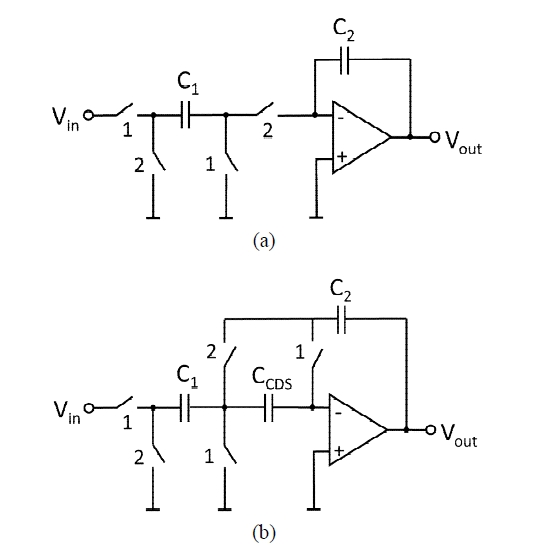For the CDS integrator, it can be easily shown that $p\cong 1-d_{0}/A_{op}^{2}$. Here, the shift of the integrator pole is greatly reduced to be proportional to $1/A_{op}^{2}$, which can be exploited in our problem to reduce the mismatch between $H_2$ and $NTF_1$. However, the gain of the CDS integrator is $d\cong d_{0}\left[1-(1+d_{0}+C_{3}/C_{2})/A_{op}\right]$, from which we observe that the reduction of the gain $d$ is still proportional to 1/$A_{op}$. Therefore, just replacing conventional integrators with CDS integrators is not enough to restore the matching. Along with the use of CDS integrators, it is necessary to adjust the loop-filter gain to compensate for the reduction of the integrator gain from finite op-amp gain. The loop-filter gain can be adjusted in many ways. The simplest one, which is used in this work, is to change the SAR ADC gain by changing the reference voltage of the ADC.

## III. BEHAVIORAL SIMULATION RESULTS

### 1. Simulated MASH ADC structure

We evaluated the performance of the proposed mismatch reduction scheme by behavioral simulations. Fig. 3 shows a block diagram of the particular MASH ADC examined in this paper. The loop-filter of the first stage is of a second order ‘cascade of integrators in feed-forward’ (CIFF) form . Table I shows the loop-filter parameters used in this work. If the op-amp gain is infinite, the NTF of the first stage is

##### (4)
\begin{equation*} \begin{array}{c} NTF_{1}\left(z\right)=\frac{\left(1-z^{-1}\right)^{2}}{1-0.25z^{-1}+0.0625z^{-2}}. \end{array} \end{equation*}

Fig. 3. Block diagram of the MASH ADC studied in this work with the loop filter structure shown in detailTable 1. Parameters for the ADC in Fig. 3

 b1 (=c1) 1 b3 1 c2 1 a1 7/4 a2 13/16

The over-sampling ratio (OSR) was 40 and an ideal SAR ADC with the total resolution ($N_{SAR}$) of 8 was assumed.

### 2. Performance with Infinite Op-amp Gain

We begin with the analysis of MASH ADC with ideal op-amps of infinite gain. Fig. 4 shows the SQNR of the ADC against the input amplitude for $N_{MSB}$’s from 1 to 4 obtained by MATLAB behavioral simulations. Fig. 4(a) shows the SQNR of the first stage output ($V_1$). We observe that a 1-bit increase of $N_{SAR}$ results in a 6 dB increase of the SQNR, as expected.

Fig. 4(b) shows the SQNR of the final output ($V$) of the two stage ADC. We can observe very large improvement of the SQNR over that of the first stage output. For $N_{SAR}$ ${\geq}$ 2, the SQNR is almost independent of $N_{SAR}$, and reaches around 110~dB. For $N_{SAR}$ = 1, the SQNR is significantly lower than that for $N_{SAR}$ ${\geq}$ 2. Furthermore, the SQNR for $N_{SAR}$ = 1 peaks at a low input amplitude of \hbox{-}15 dB. These are from the severe nonlinear behavior of the first stage delta-sigma ADC for $N_{SAR}$ = 1. In a MASH ADC, the cancellation of the first stage quantization noise by the second stage output is based on linear operation of the system. The results above show that for $N_{SAR}$ = 1, the nonlinear character of the first stage quantization noise begins to degrade the quantization noise cancellation at a very low input amplitude where it does not degrade SQNR of the first stage itself. Therefore, we conclude that $N_{SAR}$ = 1 is not suitable for the MASH ADC under study and we will not consider $N_{SAR}$ = 1 any further in the remainder of this paper.

Fig. 4. SQNR vs input amplitude for several values of $N_{SAR}$ (a) the first stage output ($V_1$), (b) the final output ($V$).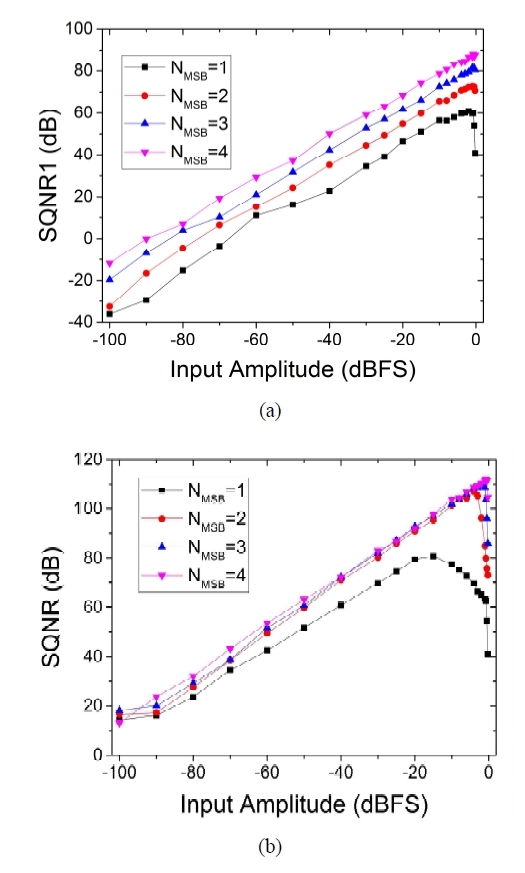### 3. Performance with Finite Op-amp Gain

Now, we investigate the effect of finite op-amp gain, which modifies the TF of the integrators by performing SPICE level behavioral simulations employing ideal analog and digital components using Spectre. Fig.~5 shows the SQNR as functions of op-amp gain for the input amplitude of -6 dB. We repeated simulations varying $N_{SAR}$ from 2 to 4. Fig. 5(a) shows the results obtained using conventional integrators. When the op-amp gain is very high, SQNR = 108~dB is achieved for all $N_{SAR}$ values. However, when the op-amp gain is reduced, the SQNR is degraded. The minimum op-amp gains required to limit the SQNR degradation within 3~dB are 69~dB, 63~dB and 57~dB for $N_{SAR}$ = 2, 3, and 4, respectively. Although it may be possible, it would require significant effort to design op-amps with such high gain with low supply voltage CMOS technologies.

Fig. 5. SQNR vs op-amp gain (a) conventional discrete-time integrators (b) CDS integrators.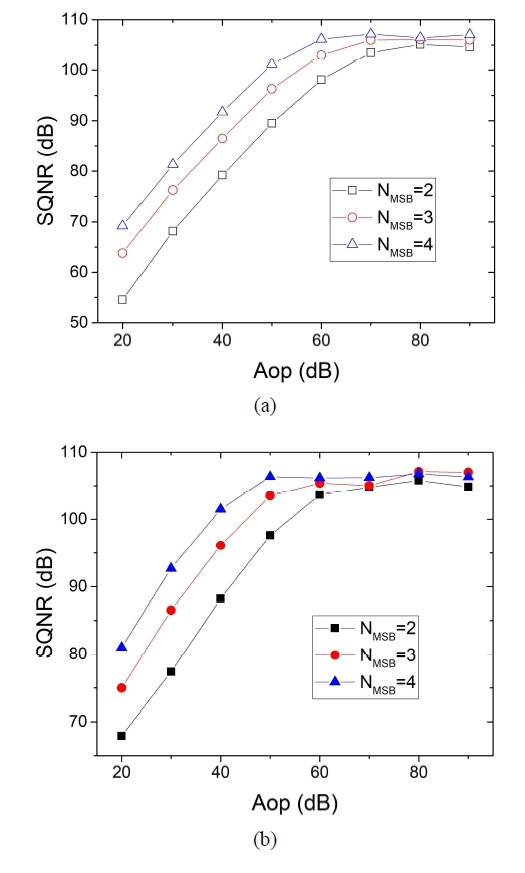Fig. 5(b) shows the simulation results obtained assuming CDS integrators. We observe that the minimum gains to limit the SQNR degradation within 3 dB are reduced to 63~dB, 53~dB, and 46~dB, respectively. Although the minimum required gains are 6 to 10 dB lower compared to those required when using conventional integrators, the improvement is not very impressive. As mentioned in Sec. II, this is because the reduction of gain $d$ of a CDS integrator is still proportional to 1/$A_{op}$, and degrades the matching between the TFs.

Now, we show that by combining simple adjustments of the quantizer gain with the employment of CDS integrators, the mismatch between TFs due to the finite op-amp gain can be greatly cancelled. Fig. 6 shows the 2D grey scale-mapping of the SQNR vs. the quantizer gain ($K_Q$) and the op-amp gain ($A_{op}$). $N_{SAR}$ = 8 and $N_{SAR}$ = 2 were used for Fig. 6. The brighter (white) region corresponds to higher SQNR and the darker region to lower SQNR.

Fig. 6. 2-dimensional gray scale mapping of SQNR vs quantizer gain ($K_Q$) and op-amp gain ($A_{op}$). $N_{SAR}$ = 8, $N_{SAR}$ = 2 (a) conventional integrators, (b) CDS integrators.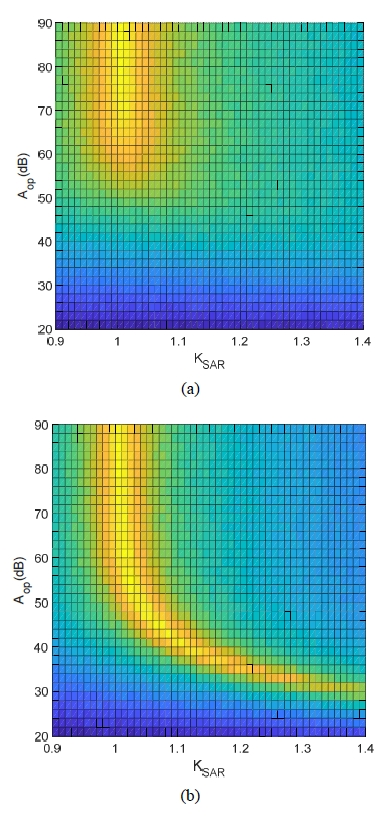Fig. 6(a) shows the results with the loop-filter employing conventional integrators. We can observe that SQNR is maximized at around $K_Q$ = 1 independent of $A_{op}$. However, as $A_{op}$ goes down below 60~dB, the bright region rapidly disappears, which means that high SQNR cannot be recovered by adjusting $K_Q$. Fig. 6(b) shows the SQNR obtained with CDS integrators. In the figure, unlike the case with conventional integrators, we can observe bright region for $A_{op}$ as low as 30~dB, although the brightness diminishes gradually for $A_{op}$ < 40 dB.

Fig. 7 shows the $K_{opt}$, which maximizes SQNR for a given $A_{op}$. We can observe that} $N_{MSB}$ = 2, 3 or 4 produces similar $K_{opt}$. Furthermore, as we already observed in Fig. 6, $K_{opt}$ approaches 1 at very large $A_{op}$, and becomes larger as $A_{op}$ is reduced. The red dashed line in Fig. 7 represent the fitting using $K_{opt}$ = 1 + 10/$A_{op}$. We can observe a very close match between the fit and the simulation results. This shows that ${\delta}K$ ${\equiv}$ $K_{opt}$ - 1 is inversely proportional to $A_{op}$, and confirms indirectly the validity of our assertion that optimizing $K_Q$ can effectively cancel the gain reduction of the integrator TF.

Fig. 8 shows the SQNR obtained by using the $K_Q$ optimized for each $A_{op}$. When we compare Fig. 8 with Fig. 5(b), which corresponds to $K_Q$ = 1, we can observe that the minimum op-amp gain requirement is greatly relaxed by optimizing $K_Q$. For example, for $N_{SAR}$ = 4, when $K_Q$ is optimized, an op-amp gain as small as 25~dB is enough to limit the SQNR degradation to less than 3~dB. When $K_Q$ = 1 was used in Fig. 5(b), the minimum required gain was 45~dB. For $N_{SAR}$ = 2, the minimum gain requirement is relaxed from 58 dB to 34 dB. These much relaxed gain requirements give circuit designers much wider design space and make the MASH ADC structure more feasible.

For this $K_Q$ optimization to be practical, the accuracy required on $K_Q$ should not be too stringent. Fig. 9 shows the SQNR improvement of the 2-stage MASH ADC over the SQNR of the first stage (${\delta}$SQNR ${\equiv}$ SQNR - SQNR$_{1}$) for several values of op-amp gain. $N_{SAR}$ = 2 was used. In Fig. 9, we observe the ranges of $K_Q$ in which ${\delta}$SQNR $>$ 30~dB are 1.08 < $K_Q$ < 1.13, 1.01 < $K_Q$ < 1.06, and 0.99 < $K_Q$ < 1.04, for $A_{op}$ = 40~dB, 50~dB, and 60~dB, respectively. These indicates that a variation of $K_Q$ larger than 5% can be accommodated. When $A_{op}$ is 30~dB, the maximum ${\delta}$SQNR itself is about 29dB. However, ${\delta}$SQNR is much less sensitive to $K_Q$ variation than when a larger $A_{op}$ is used. Therefore, if a slightly smaller SQNR improvement of ${\delta}$SQNR = 25dB is acceptable, then the accuracy of $K_Q$ should not be an issue.

Fig.8. SQNR vs $A_{op}$. Solid symbols: SQNR is maximized by optimizing $K_Q$.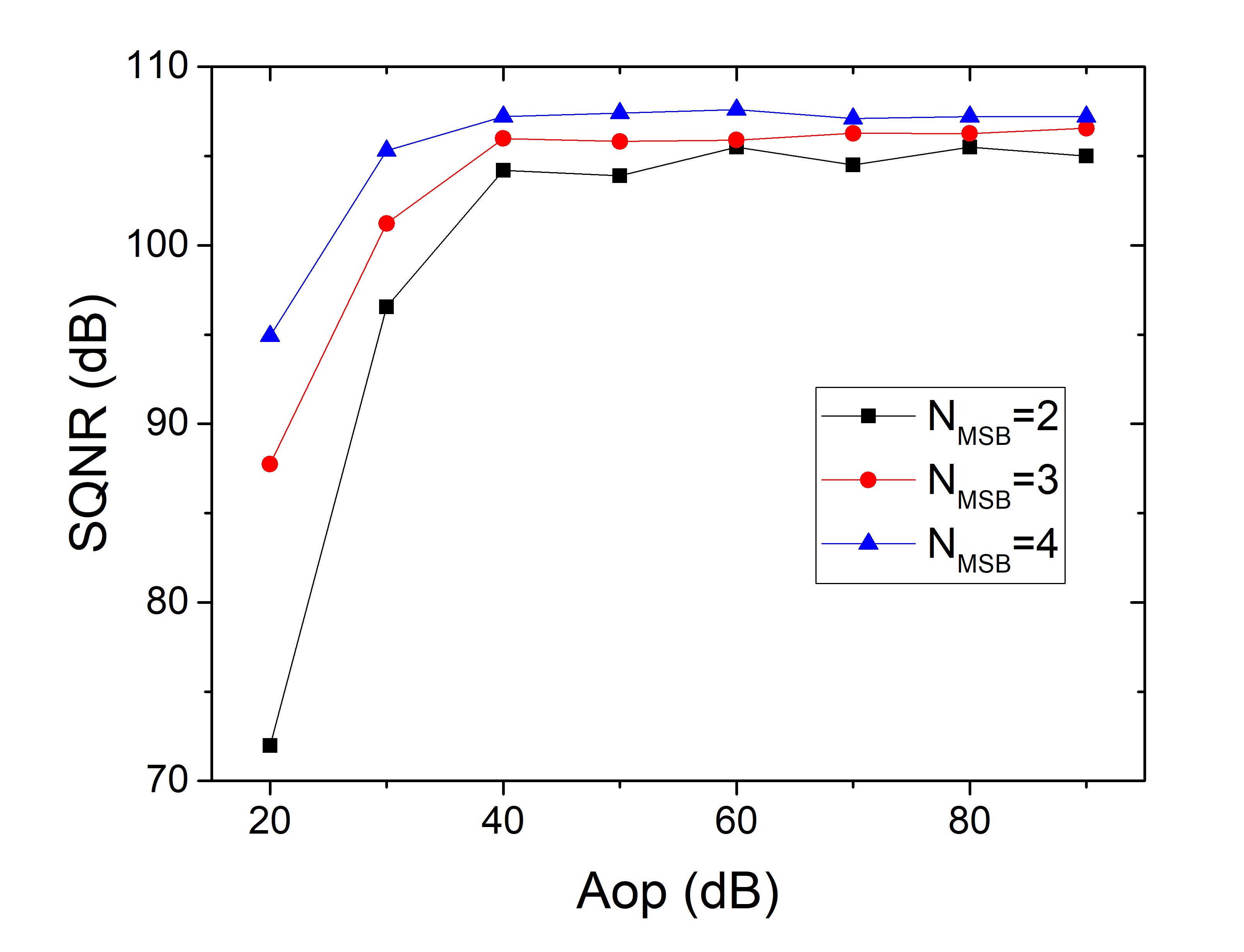Fig. 9. SQNR improvement of MASH ADC over the SQNR of the 1st-stage output vs $K_Q$. ($N_{MSB}$=2)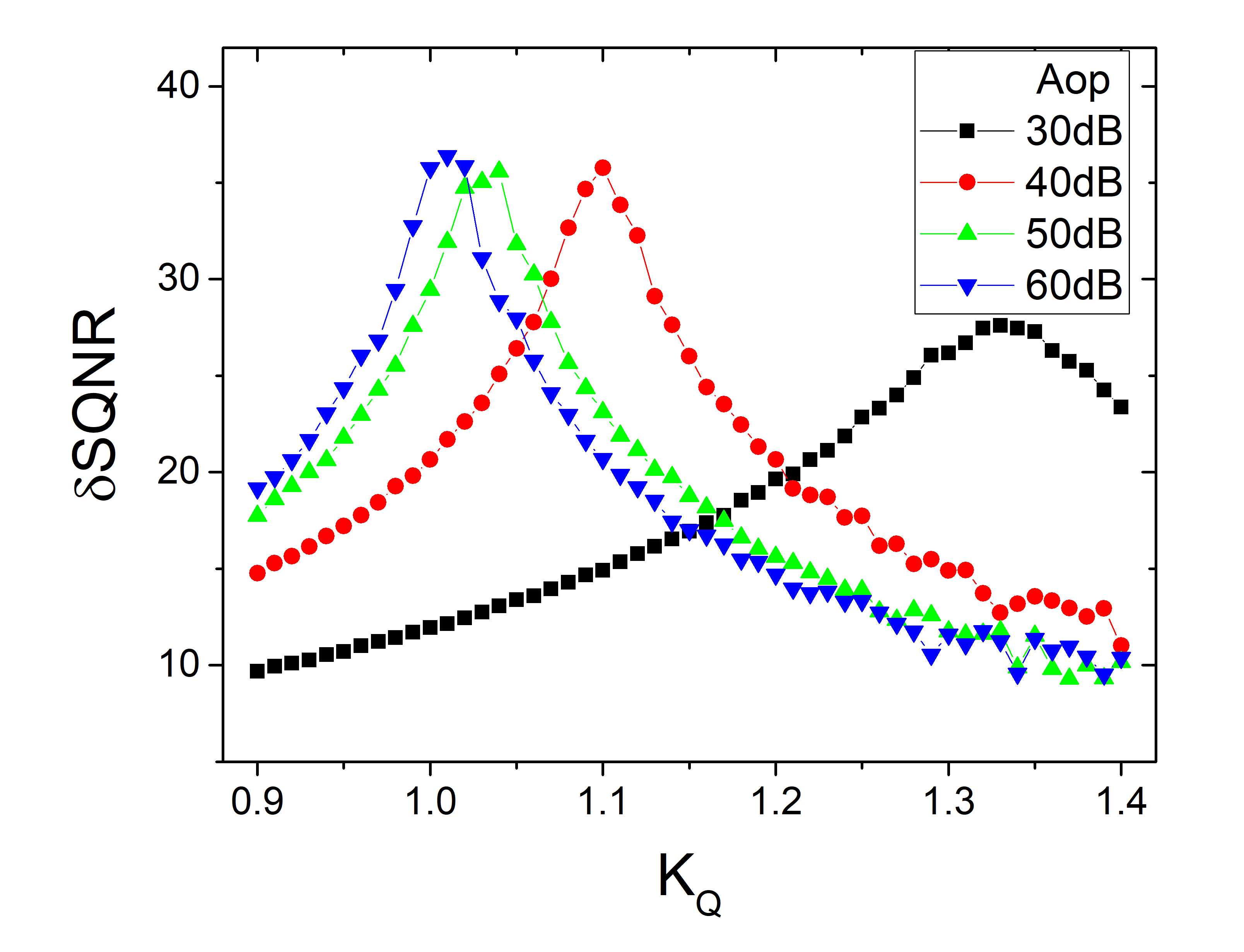In this Section, it was shown that the NTF distortion from finite op-amp gain can be compensated by optimizing the quantizer gain $K_Q$. In a SAR ADC quantizer, $K_Q$ can be adjusted easily by controlling the reference voltage. It should be noted that the same effect can be obtained by adjusting the gain parameters of the first stage loop-filter so that the input to the quantizer is scaled by the same factor.

It should also be noted that the mismatch between $NTF_1$ and $H_2$ can be cancelled in the digital domain by calibrating $H_2$. However, the digital calibration requires a multi-dimensional optimization process which might take long calibration time and/or complex logic. Furthermore, digital calibration is quite sensitive in that the filter coefficients should be optimized with a very high precision. However, our results showed that our analog domain calibration was quite insensitive to the small variation of the optimization variable $K_Q$

## IV. CONCLUSIONS

In this work, we examined the performance of a two-stage MASH ADC, in which a single SAR ADC plays the role of the quantizer for the first and the second stages. We investigated the effect of the finite op-amp gain on the degradation of the SQNR of the ADC. We found that by employing CDS integrators along with quantizer gain adjustment, we can greatly reduce the minimum required op-amp gain, which makes the realization of the MASH ADC more practical.

### ACKNOWLEDGMENTS

This work was supported by the Korea Institute for Advancement of Technology (KIAT) grant (No. N0001883, HRD Program for Intelligent semiconductor Industry) funded by the Korea Government (MOTIE), and by the R&D program of MOTIE/KEIT (10063683). The CAD tools were provided by the IC Design Education Center (IDEC), Korea.

### REFERENCES

1
Pavan S., Schreier R., Temes G. C., 2017, Understanding delta-sigma data converters, 2nd Ed., IEEE Press - Wiley, hoboken, NJ, USA2
Kwak Y.-S., Yun M.-H , Lee S.-H., Ahn G.-C., 2018, A 1.0 V 77.5 dB dynamic range delta-sigma ADC using Op-amp bias sharing technique, J. Semiconductor Technology and Science, Vol. 18, pp. 337-3453
Yang S.-H., Seong J.-H., Yoon K.-S., 2017, A reconfigurable 4th order ΣΔ modulator with a KT/C noise reduction circuit, J. Semiconductor Technology and Science, Vol. 17, pp. 294-3014
Leslie T., Singh B., May 1990, ,An improved sigma-delta modulator architecture, Proc. of IEEE Int. Symp. Circuits Syst. (ISCAS), New Orleans,LA, USA, pp. 372-3755
Shin J., Kim J., Kim S., Shin H., 2007, A delta-sigma fractional-N frequency synthesizer for quad-band multi-standard mobile broadcasting tuners in 0.18-µm CMOS, J. Semiconductor Technology and Science, Vol. 7, pp. 267-2736
Lee C. C., Alpman E., Weaver S., Lu C.-Y., Rizk J., A 66dB SNDR 15MHz BW SAR Assisted ΔΣ ADC in 22nm Tri-gate CMOS, Paper C64, Dig. Tech. Papers of 2013 Symp. VLSI Circuits7
Silva J., Moon U., Steensgaard J., Temes G. C., 2001, Wideband low-distortion delta-sigma ADC topology, Electron. Lett., Vol. 37, pp. 737-7388
Temes C., Enz C., 1996, Autozeroing, correlated double sampling, and chopper stabilization, Proc. IEEE, Vol. 84, pp. 1584-16149
Nagaraj K., Vlach J., Viswanathan T.R., Singhal K., 1986, Switched-capacitor integrator with reduced sensitivity to amplifier gain, Electron. Lett., Vol. 22, pp. 1103-1105## Author

Saemin Im received the B.S. and M.S. degrees in electronics engineering from Hanyang University, Seoul, Korea, in 2010 and 2013, respectively and is currently working towards the Ph.D. degree in the same university.

His research area is mixed-signal CMOS integrated circuits and memory interface circuits.

Jong-Yoon Shin received the B.S. and M. S. degrees in electronic engineering from Hanyang University, Seoul, Korea in 2017 and 2019, respectively.

His main research area was delta-sigma ADCs. He is currently with Samsung Electronics.

Sang-Gyu Park received B.S. and M.S. degrees in Electronics Engineering from Seoul National University in 1990 and 1992, respectively and received Ph.D. degree in Electrical and Computer Engineering from Purdue University in 1998.

He worked at AT&T Laboratories-Research from 1998 to 2000 and joined the faculty of Hanyang University in 2000, where he is a professor in Electronics and Computer Engineering.

His research area is the mixedsignal CMOS circuit design, with focus on delta-sigma oversampling data converters, high speed SAR ADCs and memory interface circuits.